# NCERT Solutions class-11 Maths Exercise 12.1## myCBSEguide App

CBSE, NCERT, JEE Main, NEET-UG, NDA, Exam Papers, Question Bank, NCERT Solutions, Exemplars, Revision Notes, Free Videos, MCQ Tests & more.

Exercise 12.1

1. A point is on the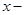axis. What are its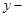coordinates and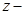coordinates?

Ans. We know that coordinates of any point on theaxis will be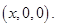Therefore,coordinate andcoordinate of the point are zero.

2. A point is on XZ-plane. What can you say about itscoordinate?

Ans. We know that coordinates of any point in XZ-plane will be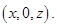Thereforecoordinate of the point is zero.

3. Name the octants in which the following points lie: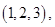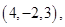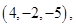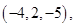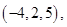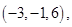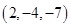Ans. Point (1, 2, 3) lies in I octant.

Point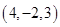lines in IV octant.

Point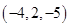lines in VIII octant.

Point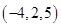lines in V octant.

Point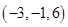lines in VIII octant.

Pointlines in VIII octant.

4. Fill in the blanks:

(i) Theaxis andaxis taken together determine a plane known as ____________.

(ii) The coordinates of points in the XY-plane are of the form ____________.

(iii) Coordinates planes divide the space into ____________ octants.

Ans. (i) XY-plane

(ii)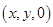(iii) Eight1. Very nice